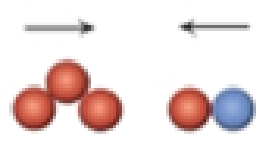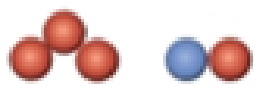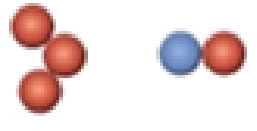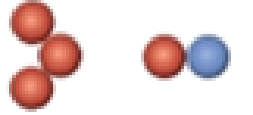Chapter 14, Problem 101SCQ

Chapter
Section
Textbook Problem

Consider the reaction of ozone and nitrogen monoxide to form nitrogen dioxide and oxygen.O3(g) + NO(g) → NO2(g) + O2(g)Which of the following orientations for the collision between ozone and nitrogen monoxide could perhaps lead to an effective collision between the molecules?(a)(b)(c)(d)Interpretation Introduction

Interpretation:

From the given set of diagrams, the diagram that fits best with the given reaction has to be determined.

Concept Introduction:

Collision theory: The rate for the reaction is predicted by using collisions theory. In order to form bond the atoms present in the reactants should collide each other for the bond formation.

In order to establish the plausibility of a mechanism, one must compare the rate law of the rate determining step to the experimentally determined rate law.

Rate determining step: In a chemical reaction the rate determining step is the slowest step in which the rate of the reaction depends on the rate of that slowest step.

Rate law: It is generally the rate equation that consists of the reaction rate with the concentration or the pressures of the reactants and constant parameters.

Activation energy: It is defined as the minimum energy required by the reacting species in order to undergo chemical reaction.

Rate constant: The rate constant for a chemical reaction is the proportionality term in the chemical reaction rate law which gives the relationship between the rate and the concentration of the reactant present in the chemical reaction.

Intermediate species: It is the species formed during the middle of the chemical reaction between the reactant and the desired product.

Explanation

Reasons for correct option:

The diagram represented in option (a) contains the reactants which are oriented linearly. The reaction is linearly oriented and the other reacting species is also linearly arranged where its end perfectly matches with the first reacting species that results to give successive collisions and pave way for the bond formation.

The species are orientated linearly in such a way that the new bond formation and the breakage of already existence is favored. Therefore, first diagram serves as the perfect orientation...

Still sussing out bartleby?

Check out a sample textbook solution.

See a sample solution

The Solution to Your Study Problems

Bartleby provides explanations to thousands of textbook problems written by our experts, many with advanced degrees!

Get Started

Can a metamorphic rock be metamorphosed?

Fundamentals of Physical Geography

Where are protons, electrons, and neutrons found in an atom?

Biology: The Dynamic Science (MindTap Course List)

Name three therapeutic uses of prostaglandins.

Chemistry for Today: General, Organic, and Biochemistry